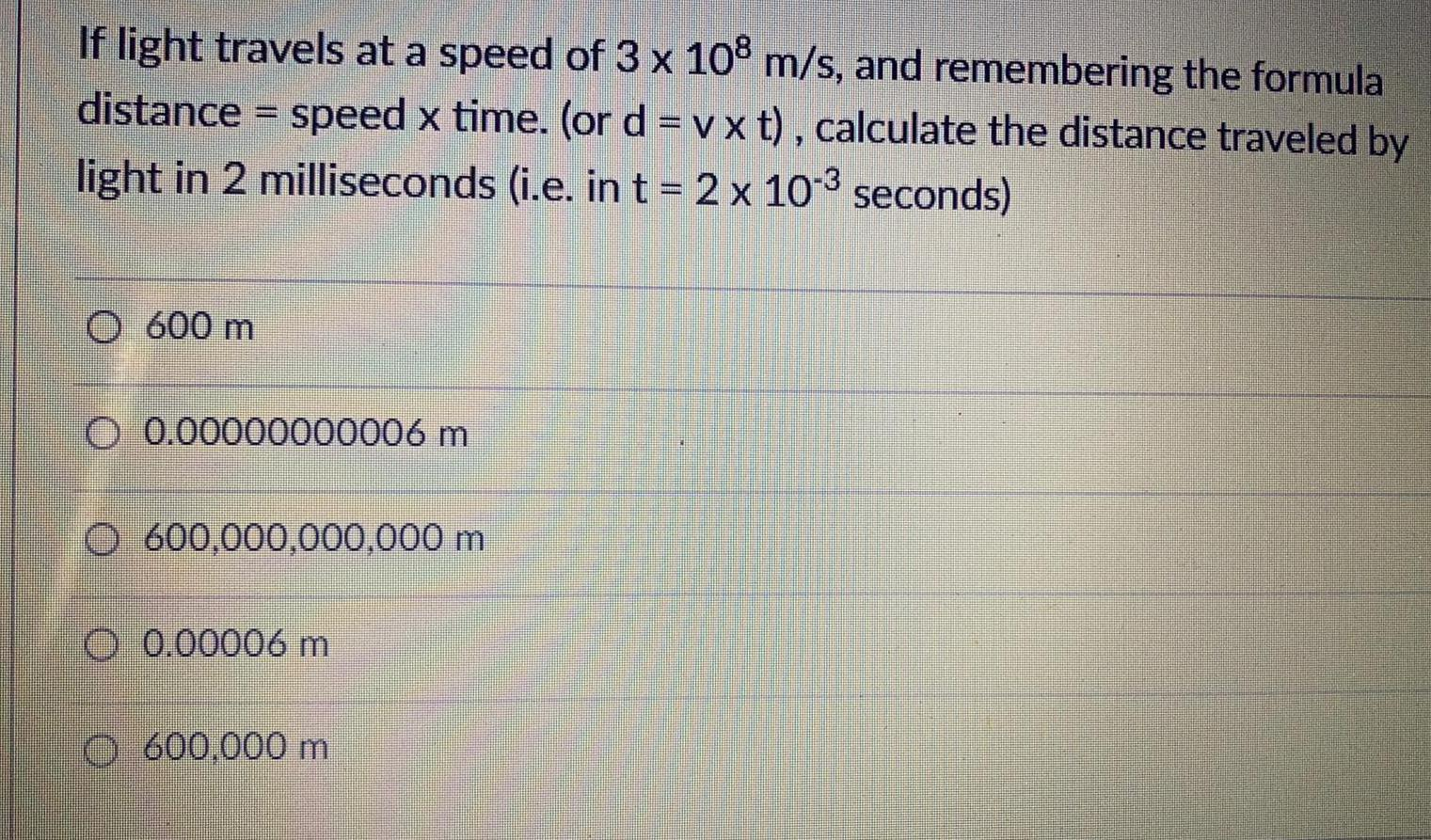Question:

# If light travels at a speed of 3 x 10⁸ m/s, and remembering

Last updated: 7/6/2022If light travels at a speed of 3 x 10⁸ m/s, and remembering the formula distance = speed x time. (or d = v*t), calculate the distance traveled by light in 2 milliseconds (i.e. in t = 2 x 10-³ seconds)